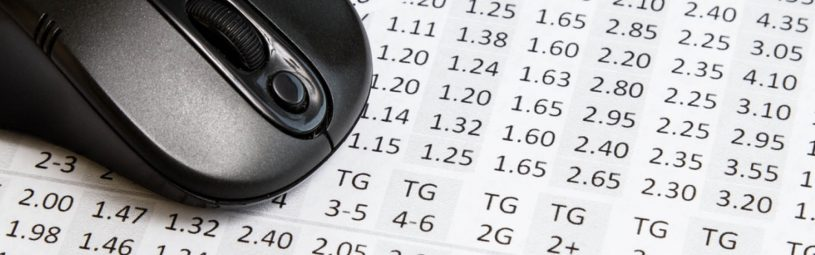# How do fractional odds work in betting?

June 19th, 2017

To the newbie punter, fractional odds must seem exactly that – odd! Yet, without odds, betting can’t actually take place and to boost your chances of backing a winner it’s important you understand them. So in this post, I’ll answer the question “how do fractional odds work?” and show you when and how to use them when placing a bet.

Read this post full a full explanation on the different types of odds that exist in sports betting.

## What are fractional odds?

Fractional odds, also known as UK Odds, are the most traditional form of odds. They indicate the probability of something happening and the amount that the bookmaker thinks that outcome is worth. Fractional odds are displayed as two numbers separated by a forward slash. Here are some examples:

5/1 – ‘five to one’ (also known as five to one against)
1/1 – ‘evens’
1/2 – ‘one to two’ (also known as two to one on)

## How do fractional odds work in betting?

Fractional odds are used to bet on sporting events and tell us two things:

1. The price – allowing you to calculate exactly how much money you will win if your bet wins.
2. Probability – the likelihood of your chosen bet actually winning.

‘Odds against’ prices. The number on the left side of the fraction shows how many times your stake will be multiplied by. For example,

• £1 stake x 5 = £5 winnings = 5/1
• £5 stake x 5 = £25 winnings = 5/1
• £10 stake x 5 = £50 winnings = 5/1

Bets placed at ‘evens’ return winnings of exactly the same amount as the stake.

• £1 stake x 1 = £1 winnings = 1/1
• £5 stake x 1 = £5 winnings = 1/1
• £10 stake x 1 = £10 winnings = 1/1

‘Odds on’ prices are calculated in reverse. The number on the right side of the fraction shows how many times your stake will be divided by. For example,

• £1 stake divided by 2 = £0.50 winnings = 1/2
• £5 stake divided by 2 = £2.50 winnings = 1/2
• £10 stake divided by 2 = £5 winnings = 1/2

## Probability

By using a fraction, you can calculate the likelihood of the outcome of an event actually happening. For example:

5/1 is calculated as: 1 / (5+1) = 0.16 or 16% probability
1/1 is calculated as: 1 / (1+1) = 0.50 or 50% probability
1/2 is calculated as: 2 / (2+1) = 0.66 or 66% probability

## Have you ever seen odds displayed as 5.0?

These are decimal odds and are an alternative format to fractional odds.There’s absolutely no difference between them. Instead, it’s just a different way to display the same information.

## Converting fractional odds to decimal

It’s really straightforward should you ever need to – simply divide the fraction and add one. For example:

5/1 as decimal odds would be 6.0
(5 divided by 1 = 5) + 1 = 6.0

15/2 as decimal odds would be 8.5
(15 divided by 2 = 7.5) + = 8.5

4/11 as decimal odds would be 1.36
(4 divided by 11 = 0.36) + 1 = 1.36

If you’re still unsure there are plenty of online odds converters you can use. Also, most bookies have settings where you can switch how odds are shown to you on their websites. Here’s an example of how to do that on Bet365:## Make sure the odds are always in your favour

Matched betting is a betting technique used to extract value from free bet offers. Bookies use promotions to entice new customers in. OddsMonkey shows you how to use this to your advantage and make real money! Matched betting eliminates the risks associated with gambling and allows you to make consistent profits without worry. Plus with OddsMonkey, you’re not on your own. Download our free Introduction to Matched Betting today to find out more.